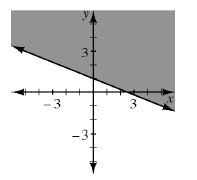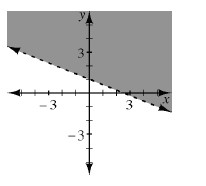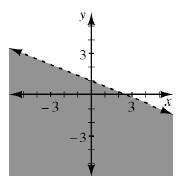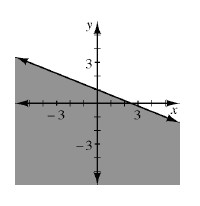### Home > AC > Chapter 10 > Lesson 10.2.5 > Problem10-98

10-98.

Multiple Choice: Which of the graphs below shows the solutions for $y < - \frac { 2 } { 5 } x + 1$?

1.1.1.1.A less than ($<$) or greater than ($>$) sign indicates a dotted line.
A less than or equal ($\le$) or greater than or equal ($\ge$) sign indicates a solid line.

(c)